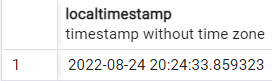# Insert Current Timestamp in PostgreSQL

Often, we have to keep the current date and time data in a database. For example, we might want to keep a log of when a customer places an order on our website.

How To Insert Current Date & Ti...
How To Insert Current Date & Time- MS Word

In such situations, when our application or website is dynamic, it is not feasible to manually write and insert the current date and time, which might also result in human errors.

Therefore, this article will discuss the different ways to insert the current timestamp in a table in PostgreSQL and their usage and limitations. Before we jump straight to them, let us first understand the meaning of timestamp.

## What Is `timestamp` in PostgreSQL

In PostgreSQL, `timestamp` is a data type that stores the date and time without a time zone. A datatype for any programming language is a specific entity distinguished by the type of values it stores.

A `timestamp` datatype variable is declared in the following way in PostgreSQL.

``````variable_name timestamp
``````

Now that you know what a `timestamp` in PostgreSQL is let us move on to understanding different functions that help us retrieve the current date and time and insert it into a `timestamp` variable.

For understanding purposes, we are creating the following table.

``````create table example1
(
variable_name timestamp
);
``````

If we want to manually insert some time and date into this table, we can do it in the following way.

``````INSERT INTO example1
VALUES ('2022-08-23 18:42:02');
``````

We can see that this data has been inserted successfully.However, as we discussed earlier, manually inserting time and date data is not a good practice. Therefore, PostgreSQL provides us with different functions which we can use to insert the current time and date in a `timestamp` variable.

Let us understand their usage one by one.

## Use the `NOW()` Function to Insert Current Timestamp in PostgreSQL

The `NOW()` function in PostgreSQL returns the current date and time with the time zone. The time zone data used is according to the settings of the database server in use.

The `NOW()` function is used in the following way.

``````SELECT NOW();
``````

We can see that the output is returning the timestamp value with the time zone as follows:If we want to convert it to a value without a time zone, we can use it in the following way.

``````SELECT NOW()::timestamp;
``````

This gives us the following output.We can use the `NOW()` function to insert the current timestamp in a table. Let us demonstrate it by inserting some values in the `example1` table.

``````INSERT INTO example1 VALUES (now());
``````

The value will be successfully inserted, as shown below.Note: The time and date value retrieved from `NOW()` will be automatically cast to `without time zone` when it is inserted in a `timestamp` variable, as shown in the output.

## Use the `CURRENT_TIMESTAMP` Function to Insert Current Timestamp in PostgreSQL

The `CURRENT_TIMESTAMP` function is similar to the `NOW()` function, as it also returns the current date and time data with the time zone.

These two functions can be used interchangeably as alternatives to each other. The `CURRENT_TIMESTAMP` function displays results in the following way.

``````SELECT CURRENT_TIMESTAMP;
``````Similar to the `NOW()` function, we can convert this data to a `timestamp` without a time zone in the following way.

``````SELECT CURRENT_TIMESTAMP::timestamp;
``````

We can see in the result that the time zone has been removed from the time data.We can use the `CURRENT_TIMESTAMP` function to insert the current timestamp in a table. Let us demonstrate it by inserting some values in the `example1` table.

``````INSERT INTO example1 VALUES (CURRENT_TIMESTAMP);
``````

The value will be successfully inserted, as shown below.Another aspect of using the `CURRENT_TIMESTAMP` function is that we can adjust the precision of the time returned. For example, we can see in the above output that the time is shown accurately to `6` decimal places.

However, PostgreSQL allows us to adjust this precision according to our needs. The syntax is demonstrated in the following query.

``````SELECT CURRENT_TIMESTAMP(2);
``````We can see in the output that the time is accurate to `2` decimal places, as requested in the precision parameter. The current timestamp can be inserted after defining a precision, as shown below.

``````INSERT INTO example1 VALUES (CURRENT_TIMESTAMP(2));
``````

The successful insertion is shown below.## Use the `LOCALTIMESTAMP` Function to Insert Current Timestamp in PostgreSQL

Lastly, we have the `LOCALTIMESTAMP` function, which serves the same purpose of retrieving the current date and time. However, unlike the `NOW()` and `CURRENT_TIMESTAMP` functions, the `LOCALTIMESTAMP` function returns time and date without a time zone.

Let us see how it is used to retrieve current timestamp data.

``````SELECT LOCALTIMESTAMP;
``````

This gives the following output.Therefore, we can use the `LOCALTIMESTAMP` function to insert the current timestamp in a table. Let us demonstrate it by inserting some values in the `example1` table.

``````INSERT INTO example1 VALUES (LOCALTIMESTAMP);
``````

The value will be successfully inserted, as shown below.Similar to the `CURRENT_TIMESTAMP` function, we can specify the time precision in the `LOCALTIMESTAMP` function. The syntax for this is as follows:

``````SELECT LOCALTIMESTAMP(2);
``````We can see in the output that the time is accurate to `2` decimal places, as requested in the precision parameter. The current timestamp can be inserted using the `LOCALTIMESTAMP` function by specifying the precision, as shown below.

``````INSERT INTO example1 VALUES (LOCALTIMESTAMP(2));
``````

The successful insertion is shown below.This sums up all the different ways to insert the current timestamp in a table in PostgreSQL. We hope you have learned how to use the `NOW()`, `CURRENT_TIMESTAMP` and `LOCALTIMESTAMP` functions to insert the date and time values in a `timestamp` datatype variable.

## Related Article - PostgreSQL Timestamp

• Convert From Unix Epoch to the Date in PostgreSQL